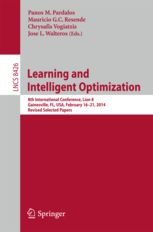• A
• A
• A
• АБB
• АБB
• АБB
• А
• А
• А
• А
• А
Обычная версия сайта

## Robust Support Vector Machines with Polyhedral Uncertainty of the Input Data

P. 291-305.
Fan N., Sadeghi E., Pardalos P. M.

In this paper, we use robust optimization models to formulate the support vector machines (SVMs) with polyhedral uncertainties of the input data points. The formulations in our models are nonlinear and we use Lagrange multipliers to give the first-order optimality conditions and reformulation methods to solve these problems. In addition, we have proposed the models for transductive SVMs with input uncertainties.

### В книгеVol. 8426. Springer, 2014.